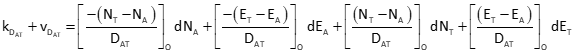## 2. DistanceFigure F-1 Distance Observation

Equation F-1 is the distance bertween two points in terms of their coordinates.Equation F-1 NA, EA: At point coordinates NT, ET: To point coordinates vD: distance residual

To apply a Taylor Series, patial derivatives of Equation F-1 are taken with respect to each coordinate of both points. The partial derivative for NA is:Similarly, the remaining partial derivatives are:Equation F-2 is the distance observation equation, evaluated at initial coordinate approximations.Equation F-2Equation F-3